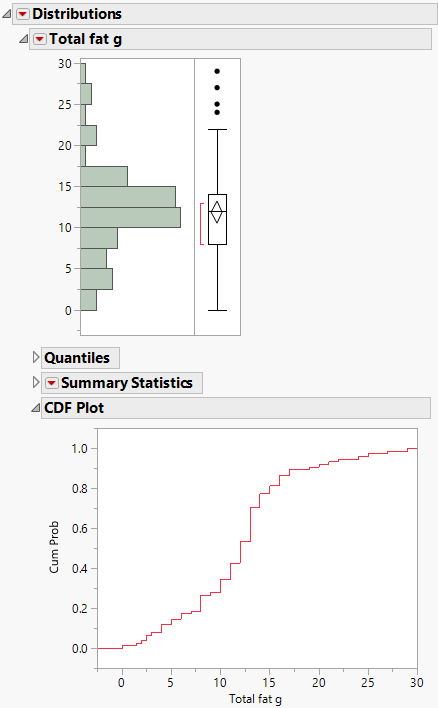Publication date: 03/23/2021

## CDF Plot

The CDF plot in Distribution creates a plot of the empirical cumulative distribution function. Use the CDF plot to determine the percent of data that is at or below a given value on the horizontal axis. When a distribution is fit to the data, the cumulative distribution function for the fitted distribution is overlaid on the CDF plot.

Figure 3.10 CDF PlotFor example, in this CDF plot, approximately 34% of the data are less than a total fat value of 10 grams.

Want more information? Have questions? Get answers in the JMP User Community (community.jmp.com).